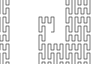http://people.csail.mit.edu/jaffer/Geometry/PSFC

# Peano Space-Filling Curves

A space-filling curve is a parameterized function which maps a unit line segment to a continuous curve in the unit square, cube, hypercube, etc, which gets arbitrarily close to a given point in the unit cube as the parameter increases.

Space-filling curves serve as a counterexample to less-than-rigorous notions of dimension. In addition to their mathematical importance, space-filling curves have applications to dimension reduction, mathematical programming [Butz 1968], multi-dimensional database indexing [Lawder 2000], and radio-frequency electronics [Zhu 2003].

The space-filling constructions are usually designed to map between the real unit line segment and the unit n-cube. My interest is to expand their range to the entirety of n-space.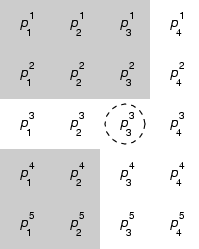### The Algorithm

In Space filling curves and mathematical programming Butz gives an algorithm for computing the Peano space-filling curve in terms of the base-3 representation of coordinates between 1 and 0. The grid shown to the right has m columns of (rank) n digits, each row corresponding to one spatial coordinate. The leftmost digits are the most significant, the ternary-point lying to their left.

Digit i of row j, pji, is replaced by 2-pji if the integer sum of:

• all the digits in columns left of column j which are not in row i and
• the digits in column j above row i
is odd. The area around digits contributing to this sum for the circled p33 is shaded in the figure.

Notice that the parity (zero vs. nonzero) of digit pji remains unchanged when it is replaced by 2-pji. Thus the order of processing digits is arbitrary. This `peano-flip!` operation is its own inverse.

A naive implementation would take time O(mn2) for the complete table. By scanning down each column from left to right while calculating the total parity and the parity for the portion of each row already visited, this can be reduced to O(mn).

Here is the simplest case. Groups of flipped digits are underlined.
scalar 0 1 2 3 4 5 6 7 8
digits
 0 0
 0 1
 0 2
 1 0
 1 1
 1 2
 2 0
 2 1
 2 2
flipped
 0 0
 0 1
 0 2
 1 2
 1 1
 1 0
 2 0
 2 1
 2 2
So within a 3*3 cell an odd higher digit flips the lower digit, creating the signature S curve.

scalar 9 10 11 12 13 14 15 16 17 18 19 20 21 22 23 24 25 26 27
digits
 0 0 1 0
 0 0 1 1
 0 0 1 2
 0 1 1 0
 0 1 1 1
 0 1 1 2
 0 2 1 0
 0 2 1 1
 0 2 1 2
 0 0 2 0
 0 0 2 1
 0 0 2 2
 0 1 2 0
 0 1 2 1
 0 1 2 2
 0 2 2 0
 0 2 2 1
 0 2 2 2
 1 0 0 0
flipped
 0 2 1 0
 0 2 1 1
 0 2 1 2
 0 1 1 2
 0 1 1 1
 0 1 1 0
 0 0 1 0
 0 0 1 1
 0 0 1 2
 0 0 2 0
 0 0 2 1
 0 0 2 2
 0 1 2 2
 0 1 2 1
 0 1 2 0
 0 2 2 0
 0 2 2 1
 0 2 2 2
 1 0 2 2
From 0 to 8 we have seen digits increment or decrement only by 1. From 8 to 9, 17 to 18, and 26 to 27 the low order digit jumps from 2 to 0 while carrying into the digit to its left.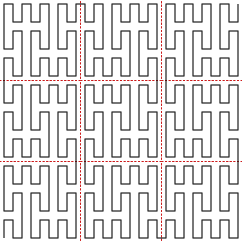### The Curve

What results is a curve with a high degree of self-similarity. Each square is composed of 9 squares; 5 of which connect bottom left to top right and 4 of which connect bottom right to top left.

The horizontal segments in the curve at right are all the same length. Vertical segments, however, are two and five times the horizontal length.

Long straight segments degrade the closeness-preserving properties important to many applications. Where a linear distance of 5 units may map to an n-space distance of 5 in this curve, in a wigglier curve it would always map to a shorter distance.

This suggests that a figure-of-merit for space-filling curves would be to sum, for every pair of m-digit points in n-space, the ratio of the euclidean distance to its corresponding linear distance. The Performance of Space-Filling Curves for Dimension Reduction reports the results of such measurements.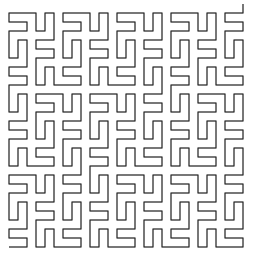### Wigglier

Each 2-dimensional cell connecting either bottom-left-to-top-right or bottom-right-to-top-left suggests a method for making the curve wigglier: swap x and y coordinates for all the bottom-left-to-top-right cells.

Generalizing this to higher ranks is not straightforward. Each 3-dimensional cell connects along one of three diagonals of the cube.

### Centering

The transforms outlined above can be easily generalized to cover nonnegative coordinates of arbitrary size by moving the ternary-point to the right. Furthermore, the precision to calculate and ternary-point position can be determined when the function is called based on the magnitude of its arguments.

Also useful would be a space-filling curve covering all integer coordinates, Zn. Although each 3mn point curve is symmetrical around its center, the coordinates calculated by the algorithm given above are nonnegative. Although the conversion from the digit table to n-space coordinates could subtract one from each digit; because the number of digits is autoscaled, discontinuities result at 3mn boundaries.

More fundamental is that, if all of n-space is to be covered by a double-ended self-similar curve, then negative linear numbers must paint half of it. Thus the positive half of linear numbers in the range -3mn/2 to 3mn/2 must paint a shape other than a square. Fortunately, they form what could be described as a rectilinear spiral: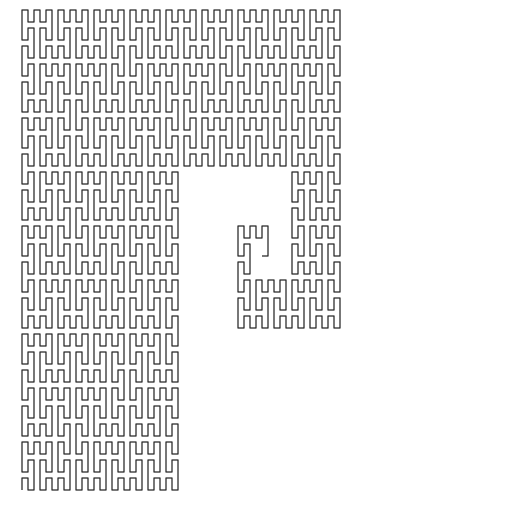The algorithm to convert from an integer scalar to n centered Peano coordinates is:

• Find the smallest m such that 9mn/2 is greater or equal to absolute-value of scalar;
• Convert scalar + 9mn/2 into a ternary digit array;
• `peano-flip!` the ternay digit array;
• extract each coordinate from its array row; offsetting it by -9m/2.

The algorithm to convert from n centered Peano coordinates to an integer scalar is:

• Find the smallest m such that 9m/2 is greater or equal to the absolute-value of each the input coordinate.
• Convert the coordinates offset by +9m/2 into a ternary digit array;
• `peano-flip!` the ternay digit array;
• extract the scalar from the array; offsetting it by -9mn/2.

The divisions all round toward zero. The base of the exponents is 9 rather than 3 because, although the center square of the 3m scale cube has the same orientation as the corner squares, it is traversed in the reverse direction.

### Praxis

slib/peanosfc.scm is a Scheme implementation of Peano conversions between integer scalars and integer coordinate vectors of arbitrary size.

Function: natural->peano-coordinates scalar rank

Returns a list of rank nonnegative integer coordinates corresponding to exact nonnegative integer scalar. The lists returned by `natural->peano-coordinates` for scalar arguments 0 and 1 will differ in the first element.

Function: peano-coordinates->natural coords

Returns an exact nonnegative integer corresponding to coords, a list of nonnegative integer coordinates.

Function: integer->peano-coordinates scalar rank

Returns a list of rank integer coordinates corresponding to exact integer scalar. The lists returned by `integer->peano-coordinates` for scalar arguments 0 and 1 will differ in the first element.

Function: peano-coordinates->integer coords

Returns an exact integer corresponding to coords, a list of integer coordinates.

### Further Work

• Develop a "wigglier" variant of the Peano curve.

The Peano curve described here does not have the isotropy property called for by Multidimensional Space-Filling Curves:

the lengths of axis-aligned projections of the space-filling curve should be equal.
The Peano curve reflects cells in order to make them contiguous, but does not perform the rotation causing such vexation in Recursive Formulation of Multidimensional Space-Filling Functions

It should be possible to rotate those cells currently subject to an odd number of reflections. That would rotate 4 of the 9 subcells of each cell.

• Write comparison functions for Peano fixed-point coordinates.

• Write comparison functions for Peano floating-point coordinates.

• Use these functions to extend the WB B-trees to multidimensional indexes a la [Lawder 2000].